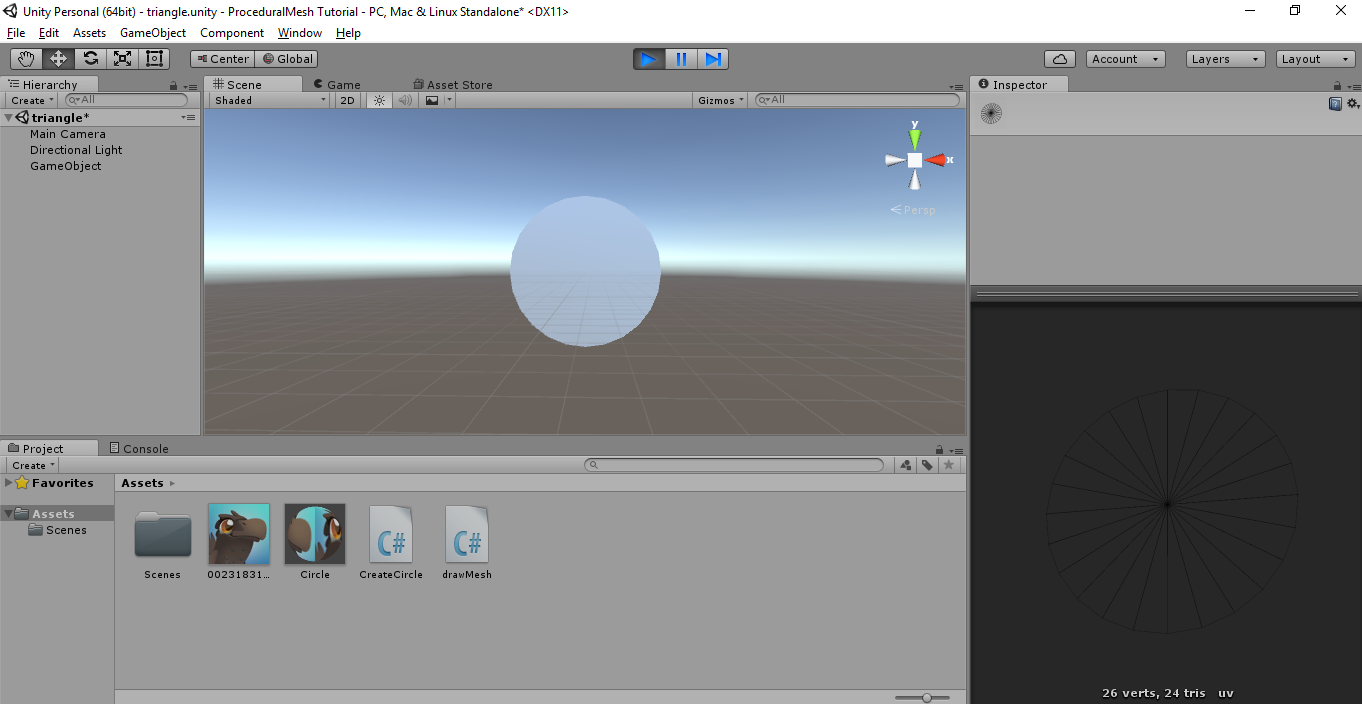#### Procedural Mesh in Unity, Part-2 Lets Draw A Circle

01 Sep 2017 | Sujay#### What are we up to?

In my previous article, we learned how to generate a procedural mesh for a triangle in Unity. In this post, I will write a slightly complicated mesh, a circle.

#### The computer graphics perspective of a circle:

We know that any object in CG is essentially made out of triangles and quads. But how do we draw a circle using triangles?? Triangles are made out of straight faces. How do we draw curves using only triangles?

Observe any polygon that has more than 5 sides. The more the number of sides a polygon has, the more it looks like a circle. If we increase the number of sides in the polygon to infinity you have an ideal circle. But computers are finite machines. We cant draw infinite triangles just to get a mere circle. So, let’s limit the number of sides to say 24, which is just enough to make the polygon look like a circle. Basically, we draw a circle as a polygon with a large number of sides, which is definitely possible to draw with triangles.

#### Mathematical perspective of a circle:

We saw the CG perspective of the circle as a polygon with a large number of sides. Now let us try to understand the circle from a mathematical perspective. A circle with radius r and center (h,k) can be defined by a following Cartesian equation.

If we take origin as the center, then the equation reduces to

If we know the radius of the circle, we have 2 variables left. We can try to plug in all the possible values for x and y and see if they lie on the given circle. But this is computationally intensive. Instead, there is a better approach. In CG we mostly use the spherical coordinate system. In spherical coordinates, the circle can be defined as follows.

Where theta is the angle between 00 and 3600. To draw a circle from this equation, all we need to do is have a constant radius, and sweep the angle theta across all the possible values between 00 and 3600. This will give us all the points that lie on the circumference of the circle. This is way better than the previous approach.

#### Writing the script:

Once we have the mathematical equation of the circle all that is left is translating the equation to a set of instructions your dumb machine can understand.

Let us first define few variables.

The first variable val that we will use for converting the theta from degrees to radians. We need a radius for our circle. Let us define a variable to hold the step value. It is better to choose a number that divides 360. Then let us choose the origin as the center of the triangle.

Let us add the center of the circle into our vertices array. Note that this vertex is going to be a common vertex for all the triangles that make up this circle. Lets also find the first point (point1) on the circumference at theta=0. We also map its UVs.

Now we should start finding other points on the circumference of the circle.

What we are doing here is sweeping theta from 0 to 360 in steps of deltaAngle. At each step, we find the point that lies on the circumference and stores that point to the vertices list. We will use a variable to keep track of the number of triangles that we draw. This variable is used in defining the triangles. As explained earlier each triangle has one common vertex, that is the center of the circle. The other 2 points would be the newly found point and the previously found point. Also, it is important to define the triangles in clockwise order.

I have added the UV mapping here. This is important if you want to add an image overlay on top of the mesh. The UVs are mapped in a linear order from top left to bottom right. Finally increase the triangle count, also the current point must be assigned to point1 as it will be referred to in the next iteration.

Add the obtained lists to the mesh. Save the script and run the scene in the editor. You should see the circle in your scene.

Try playing around with the values like radius and deltaAngle to get the circle for your use case. You will get a smoother circle as you decrease the deltaAngle.

The code for this tutorial along with other procedural meshes in this series is available here.